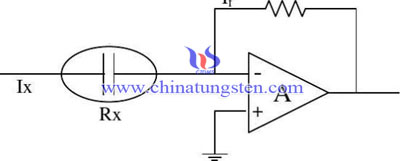# 钨粒测试碳硫含量 - 电导法4FeS+7O2=2Fe2O3+4SO2
2Fe3C+5O2=6FeO+2CO2
SO2+H2O=H2SO3
3H2SO3+K2Cr2+H2SO4=Cr2(SO4)3+K2SO4+4H2O
CO2+2NaOH=Na2CO3+H2O